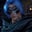Related Tags

python3
communitycreator

# Fizz Buzz implementation in PythonGutha Vamsi Krishna

### Problem

Write a program that prints numbers from 1 to 100, and:

• For multiples of 3, print Fizz instead of the number.
• For multiples of 5, print Buzz.
• For multiples of 3 and 5, print FizzBuzz.
• For others, print number.

### Example

#### Input

1,2,3,4,5... 100

#### Output

1, 2, Fizz, 4, Buzz, Fizz, 7, 8, Fizz, Buzz, 11, Fizz, 13, 14, FizzBuzz

#### Explanation

• 1 and 2 are not multiples of either 3 or 5, so we print the same number.

• 3 is a multiple of 3, so we print Fizz.

• 5 is a multiple of 5, so we print Buzz.

• 6 is a multiple of 3, so we print Fizz.

• 7 and 8 are not multiples of either 3 or 5, so we print the same number.

• 9 is a multiple of 3, so we print Fizz.

• 10 is a multiple of 5, so we print Fizz.

• 11 is not a multiple of either 3 or 5, so we print the same number.

• 12 is a multiple of 3, so we print Fizz.

• 13 and 14 are not multiples of either 3 or 5, so we print the same number.

• 15 is a multiple of both 3 and 5, so we print FizzBuzz.

### Implementation in Python

We will use a for-in-range loop to solve this problem.

In the code snippet below, we use a for-in-range loop to traverse numbers from 1 to 100.

Note that we use 101; the loop won’t include last element, so it goes up to 100.

Use if-elif-else to check if the number is divisible by 3 or 5 or both.

• Check with both the numbers and then check for the numbers individually, as shown in the code below.
• If the number is not divisible by neither 3 nor 5, then print the same number.
#for loop that traverses numbers from 1 to 100
for i in range(1,101):
#check if number is divisible by both 3 and 5
if(i%3==0 and i%5==0):
print("FizzBuzz")
#check if number is divisible by 3
elif(i%3 == 0):
print("Fizz")
#check if number is divisible by 5
elif(i%5 == 0):
print("Buzz")
#if not divisible by either of them print the i
else:
print(i)

RELATED TAGS

python3
communitycreator

CONTRIBUTORGutha Vamsi Krishna
RELATED COURSES

View all Courses

Keep Exploring

Learn in-demand tech skills in half the time